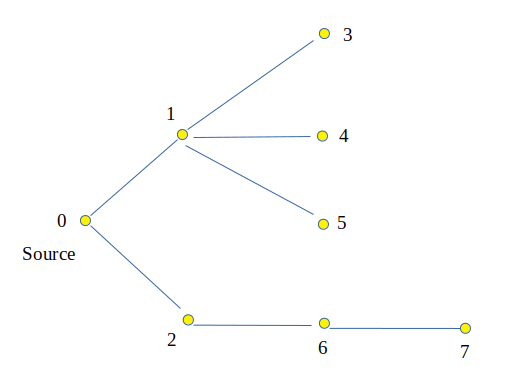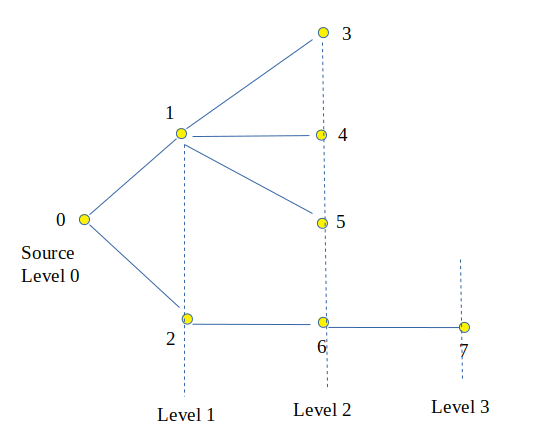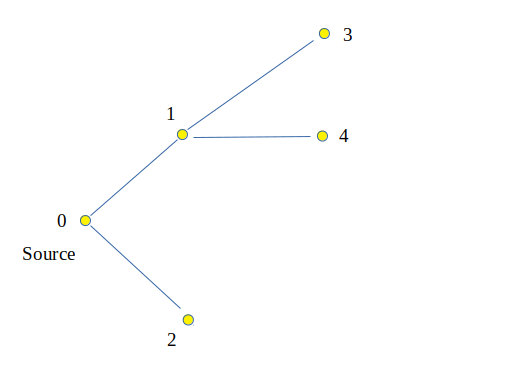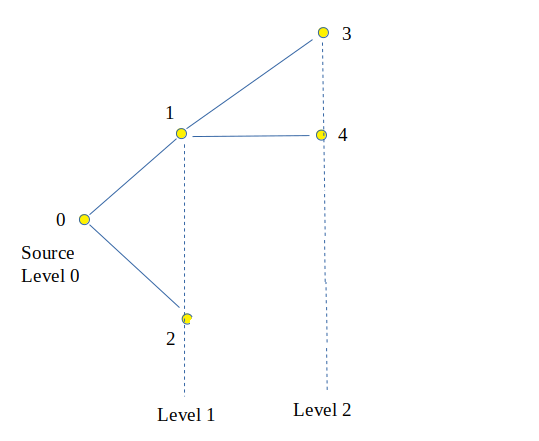# Level of Each node in a Tree from source node (using BFS)

Given a tree with v vertices, find the level of each node in a tree from the source node.

Examples:

```Input :Output :  Node      Level
0          0
1          1
2          1
3          2
4          2
5          2
6          2
7          3

Explanation :Input:Output :  Node      Level
0          0
1          1
2          1
3          2
4          2
Explanation:```

Approach:
BFS(Breadth-First Search) is a graph traversal technique where a node and its neighbours are visited first and then the neighbours of neighbours. In simple terms, it traverses level-wise from the source. First, it traverses level 1 nodes (direct neighbours of source node) and then level 2 nodes (neighbours of source node) and so on. The BFS can be used to determine the level of each node from a given source node.

Algorithm:

1. Create the tree, a queue to store the nodes and insert the root or starting node in the queue. Create an extra array level of size v (number of vertices) and create a visited array.
2. Run a loop while size of queue is greater than 0.
3. Mark the current node as visited.
4. Pop one node from the queue and insert its childrens (if present) and update the size of the inserted node as level[child] = level[node] + 1.
5. Print all the node and its level.

Implementation:

## C++

 `// CPP Program to determine level of each node ` `// and print level ` `#include ` `using` `namespace` `std; ` ` `  `// function to determine level of each node starting ` `// from x using BFS ` `void` `printLevels(vector<``int``> graph[], ``int` `V, ``int` `x) ` `{ ` `    ``// array to store level of each node ` `    ``int` `level[V]; ` `    ``bool` `marked[V]; ` ` `  `    ``// create a queue ` `    ``queue<``int``> que; ` ` `  `    ``// enqueue element x ` `    ``que.push(x); ` ` `  `    ``// initialize level of source node to 0 ` `    ``level[x] = 0; ` ` `  `    ``// marked it as visited ` `    ``marked[x] = ``true``; ` ` `  `    ``// do until queue is empty ` `    ``while` `(!que.empty()) { ` ` `  `        ``// get the first element of queue ` `        ``x = que.front(); ` ` `  `        ``// dequeue element ` `        ``que.pop(); ` ` `  `        ``// traverse neighbors of node x ` `        ``for` `(``int` `i = 0; i < graph[x].size(); i++) { ` `            ``// b is neighbor of node x ` `            ``int` `b = graph[x][i]; ` ` `  `            ``// if b is not marked already ` `            ``if` `(!marked[b]) { ` ` `  `                ``// enqueue b in queue ` `                ``que.push(b); ` ` `  `                ``// level of b is level of x + 1 ` `                ``level[b] = level[x] + 1; ` ` `  `                ``// mark b ` `                ``marked[b] = ``true``; ` `            ``} ` `        ``} ` `    ``} ` ` `  `    ``// display all nodes and their levels ` `    ``cout << ``"Nodes"` `         ``<< ``"    "` `         ``<< ``"Level"` `<< endl; ` `    ``for` `(``int` `i = 0; i < V; i++) ` `        ``cout << ``" "` `<< i << ``"   -->   "` `<< level[i] << endl; ` `} ` ` `  `// Driver Code ` `int` `main() ` `{ ` `    ``// adjacency graph for tree ` `    ``int` `V = 8; ` `    ``vector<``int``> graph[V]; ` ` `  `    ``graph.push_back(1); ` `    ``graph.push_back(2); ` `    ``graph.push_back(3); ` `    ``graph.push_back(4); ` `    ``graph.push_back(5); ` `    ``graph.push_back(5); ` `    ``graph.push_back(6); ` `    ``graph.push_back(7); ` ` `  `    ``// call levels function with source as 0 ` `    ``printLevels(graph, V, 0); ` ` `  `    ``return` `0; ` `} `

## Java

 `// Java Program to determine level of each node  ` `// and print level  ` `import` `java.util.*; ` ` `  `class` `GFG ` `{ ` `     `  `// function to determine level of each node starting  ` `// from x using BFS  ` `static` `void` `printLevels(Vector> graph, ``int` `V, ``int` `x)  ` `{  ` `    ``// array to store level of each node  ` `    ``int` `level[] = ``new` `int``[V];  ` `    ``boolean` `marked[] = ``new` `boolean``[V];  ` ` `  `    ``// create a queue  ` `    ``Queue que = ``new` `LinkedList();  ` ` `  `    ``// enqueue element x  ` `    ``que.add(x);  ` ` `  `    ``// initialize level of source node to 0  ` `    ``level[x] = ``0``;  ` ` `  `    ``// marked it as visited  ` `    ``marked[x] = ``true``;  ` ` `  `    ``// do until queue is empty  ` `    ``while` `(que.size() > ``0``)  ` `    ``{  ` ` `  `        ``// get the first element of queue  ` `        ``x = que.peek();  ` ` `  `        ``// dequeue element  ` `        ``que.remove();  ` ` `  `        ``// traverse neighbors of node x  ` `        ``for` `(``int` `i = ``0``; i < graph.get(x).size(); i++)  ` `        ``{  ` `            ``// b is neighbor of node x  ` `            ``int` `b = graph.get(x).get(i);  ` ` `  `            ``// if b is not marked already  ` `            ``if` `(!marked[b]) ` `            ``{  ` ` `  `                ``// enqueue b in queue  ` `                ``que.add(b);  ` ` `  `                ``// level of b is level of x + 1  ` `                ``level[b] = level[x] + ``1``;  ` ` `  `                ``// mark b  ` `                ``marked[b] = ``true``;  ` `            ``}  ` `        ``}  ` `    ``}  ` ` `  `    ``// display all nodes and their levels  ` `    ``System.out.println( ``"Nodes"` `                        ``+ ``" "` `                        ``+ ``"Level"``);  ` `    ``for` `(``int` `i = ``0``; i < V; i++)  ` `        ``System.out.println(``" "` `+ i +``" --> "` `+ level[i] );  ` `}  ` ` `  `// Driver Code  ` `public` `static` `void` `main(String args[]) ` `{  ` `    ``// adjacency graph for tree  ` `    ``int` `V = ``8``;  ` `    ``Vector> graph=``new` `Vector>();  ` `     `  `    ``for``(``int` `i = ``0``; i < V + ``1``; i++) ` `    ``graph.add(``new` `Vector()); ` ` `  `    ``graph.get(``0``).add(``1``);  ` `    ``graph.get(``0``).add(``2``);  ` `    ``graph.get(``1``).add(``3``);  ` `    ``graph.get(``1``).add(``4``);  ` `    ``graph.get(``1``).add(``5``);  ` `    ``graph.get(``2``).add(``5``);  ` `    ``graph.get(``2``).add(``6``);  ` `    ``graph.get(``6``).add(``7``);  ` ` `  `    ``// call levels function with source as 0  ` `    ``printLevels(graph, V, ``0``);  ` `}  ` `}  ` ` `  `// This code is contributed by Arnab Kundu `

## Python3

 `# Python3 Program to determine level  ` `# of each node and print level  ` `import` `queue  ` ` `  `# function to determine level of  ` `# each node starting from x using BFS  ` `def` `printLevels(graph, V, x): ` `     `  `    ``# array to store level of each node  ` `    ``level ``=` `[``None``] ``*` `V  ` `    ``marked ``=` `[``False``] ``*` `V  ` ` `  `    ``# create a queue  ` `    ``que ``=` `queue.Queue() ` ` `  `    ``# enqueue element x  ` `    ``que.put(x)  ` ` `  `    ``# initialize level of source  ` `    ``# node to 0  ` `    ``level[x] ``=` `0` ` `  `    ``# marked it as visited  ` `    ``marked[x] ``=` `True` ` `  `    ``# do until queue is empty  ` `    ``while` `(``not` `que.empty()): ` ` `  `        ``# get the first element of queue  ` `        ``x ``=` `que.get()  ` ` `  `        ``# traverse neighbors of node x ` `        ``for` `i ``in` `range``(``len``(graph[x])): ` `             `  `            ``# b is neighbor of node x  ` `            ``b ``=` `graph[x][i]  ` ` `  `            ``# if b is not marked already  ` `            ``if` `(``not` `marked[b]):  ` ` `  `                ``# enqueue b in queue  ` `                ``que.put(b)  ` ` `  `                ``# level of b is level of x + 1  ` `                ``level[b] ``=` `level[x] ``+` `1` ` `  `                ``# mark b  ` `                ``marked[b] ``=` `True` ` `  `    ``# display all nodes and their levels  ` `    ``print``(``"Nodes"``, ``" "``, ``"Level"``) ` `    ``for` `i ``in` `range``(V): ` `        ``print``(``" "``,i,  ``" --> "``, level[i]) ` ` `  `# Driver Code  ` `if` `__name__ ``=``=` `'__main__'``: ` ` `  `    ``# adjacency graph for tree  ` `    ``V ``=` `8` `    ``graph ``=` `[[] ``for` `i ``in` `range``(V)] ` ` `  `    ``graph[``0``].append(``1``)  ` `    ``graph[``0``].append(``2``)  ` `    ``graph[``1``].append(``3``)  ` `    ``graph[``1``].append(``4``)  ` `    ``graph[``1``].append(``5``)  ` `    ``graph[``2``].append(``5``)  ` `    ``graph[``2``].append(``6``)  ` `    ``graph[``6``].append(``7``)  ` ` `  `    ``# call levels function with source as 0  ` `    ``printLevels(graph, V, ``0``) ` ` `  `# This code is contributed by PranchalK `

## C#

 `// C# Program to determine level of each node  ` `// and print level  ` `using` `System; ` `using` `System.Collections.Generic; ` ` `  `class` `GFG ` `{ ` `     `  `// function to determine level of each node starting  ` `// from x using BFS  ` `static` `void` `printLevels(List> graph,  ` `                                  ``int` `V, ``int` `x)  ` `{  ` `    ``// array to store level of each node  ` `    ``int` `[]level = ``new` `int``[V];  ` `    ``Boolean []marked = ``new` `Boolean[V];  ` ` `  `    ``// create a queue  ` `    ``Queue<``int``> que = ``new` `Queue<``int``>();  ` ` `  `    ``// enqueue element x  ` `    ``que.Enqueue(x);  ` ` `  `    ``// initialize level of source node to 0  ` `    ``level[x] = 0;  ` ` `  `    ``// marked it as visited  ` `    ``marked[x] = ``true``;  ` ` `  `    ``// do until queue is empty  ` `    ``while` `(que.Count > 0)  ` `    ``{  ` ` `  `        ``// get the first element of queue  ` `        ``x = que.Peek();  ` ` `  `        ``// dequeue element  ` `        ``que.Dequeue();  ` ` `  `        ``// traverse neighbors of node x  ` `        ``for` `(``int` `i = 0; i < graph[x].Count; i++)  ` `        ``{  ` `            ``// b is neighbor of node x  ` `            ``int` `b = graph[x][i];  ` ` `  `            ``// if b is not marked already  ` `            ``if` `(!marked[b]) ` `            ``{  ` ` `  `                ``// enqueue b in queue  ` `                ``que.Enqueue(b);  ` ` `  `                ``// level of b is level of x + 1  ` `                ``level[b] = level[x] + 1;  ` ` `  `                ``// mark b  ` `                ``marked[b] = ``true``;  ` `            ``}  ` `        ``}  ` `    ``}  ` ` `  `    ``// display all nodes and their levels  ` `    ``Console.WriteLine(``"Nodes"` `+ ``" "` `+ ``"Level"``);  ` `    ``for` `(``int` `i = 0; i < V; i++)  ` `        ``Console.WriteLine(``" "` `+ i +``" --> "` `+ level[i]);  ` `}  ` ` `  `// Driver Code  ` `public` `static` `void` `Main(String []args) ` `{  ` `    ``// adjacency graph for tree  ` `    ``int` `V = 8;  ` `    ``List> graph = ``new` `List>();  ` `     `  `    ``for``(``int` `i = 0; i < V + 1; i++) ` `        ``graph.Add(``new` `List<``int``>()); ` ` `  `    ``graph.Add(1);  ` `    ``graph.Add(2);  ` `    ``graph.Add(3);  ` `    ``graph.Add(4);  ` `    ``graph.Add(5);  ` `    ``graph.Add(5);  ` `    ``graph.Add(6);  ` `    ``graph.Add(7);  ` ` `  `    ``// call levels function with source as 0  ` `    ``printLevels(graph, V, 0);  ` `}  ` `} ` ` `  `// This code is contributed by Princi Singh `

Output:

```Nodes    Level
0   -->   0
1   -->   1
2   -->   1
3   -->   2
4   -->   2
5   -->   2
6   -->   2
7   -->   3
```

Complexity Analysis:

• Time Complexity: O(n).
In BFS traversal every node is visited only once, so Time Complexity is O(n).
• Space Complexity: O(n).
The space is required to store the nodes in a queue.

Attention reader! Don’t stop learning now. Get hold of all the important DSA concepts with the DSA Self Paced Course at a student-friendly price and become industry ready.

My Personal Notes arrow_drop_upCheck out this Author's contributed articles.

If you like GeeksforGeeks and would like to contribute, you can also write an article using contribute.geeksforgeeks.org or mail your article to contribute@geeksforgeeks.org. See your article appearing on the GeeksforGeeks main page and help other Geeks.

Please Improve this article if you find anything incorrect by clicking on the "Improve Article" button below.

Article Tags :
Practice Tags :

9

Please write to us at contribute@geeksforgeeks.org to report any issue with the above content.